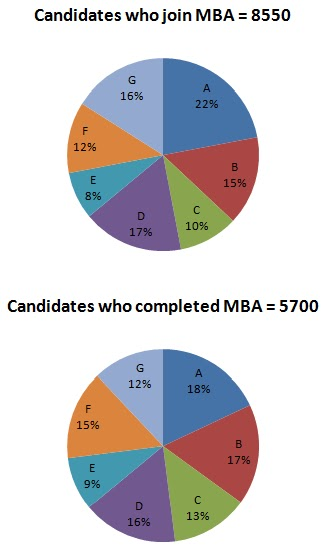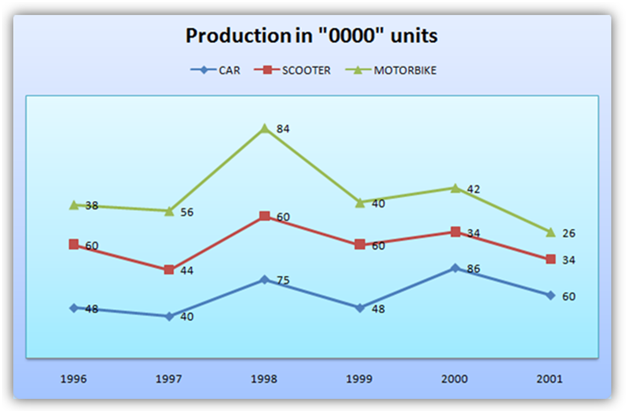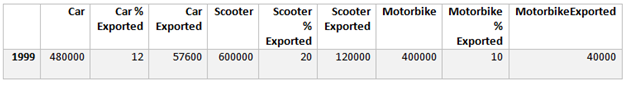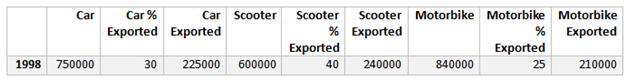Data Interpretation Practice Quiz for SBI PO and NIACL Mains

Data Interpretation Quiz for SBI PO and NIACL Mains
Directions (1 – 5): Study the following Pie charts and answer the questions followed
Distribution of Candidates who joined M.B.A. Programme and successfully completed1. Which institute has the highest percentage of candidates who completed the M.B.A programme out of the candidates that joined?
(a) C
(b) B
(c) F
(d) E
(e) A
2. What percentage of candidates completed the M.B.A. programme from Institute E out of the total number of candidates that joined the same institute?
(a) 62.5%
(b) 60%
(c) 75%
(d) 50%
(e) 62.75%
3. What is the ratio of candidates who successfully completed the M.B.A. programme to the candidates who joined from Institute G?
(a) 3 : 4
(b) 1 : 2
(c) 6 : 11
(d) 9 : 17
(e) 6 : 13
4. What percentage of candidates completed the M.B.A. programme out of the candidates who joined for Institutes Band C together?
(a) 64%
(b) 75%
(c) 90%
(d) 80%
(e) 78%
5. The number of candidates who completed the M.B.A. programme from A and D Institutes together exceeds the number of candidates who joined Institutes C and E together by
(a) 304
(b) 278
(c) 399
(d) 369
(e) 402
Directions (6 – 10): Study the following line graphs which show the production (in ‘0000 units) and percentage export of scooters, motorbikes and car respectively over the years.6. In which of the following years was the production of cars more than 50% of the total production?
(a) 2000
(b) 2001
(c) 1998
(d) 1996
(e) 1997
7. Find the total number of automobiles exported in the year 1999.
(a) 227600
(b) 207600
(c) 217600
(d) 220000
(e) 23600
8. Find the ratio of cars, scooters and motorbikes exported in 1996.
(a) 25 : 16 : 19
(b) 16 : 25 : 19
(c) 19 : 16 : 25
(d) 6 : 5 : 1
(e) 7 : 9 : 1
9. If the ratio of export prices of a cars, scooter and motorbike was 2 : 1 : 1.5 in 1998, what was the proportion of their export earnings?
(a) 4 : 2 : 3
(b) 6 : 1 : 21
(c) 30: 16: 21
(d) Cannot be determined
(e) None of these
10. In which of the following years was the production of motorbikes exactly 40% of the total production of automobiles in that years?
(a) 1997
(b) 2000
(c) 1999
(d) 1996
(e) 2001
Solutions:
1: (a)
Since the ratio of total candidates who joined MBA to the total students
who completed will be constant throughout, only the percentage value ratios need to be considered.
For C we have the ratio 13/10 = 1.3
For B we have the ratio 17/15 = 1.13
For F we have the ratio 15/12 = 1.25
For E we have the ratio 9/8 = 1.125
As the ratio is maximum for C, so C has the highest  percentage of candidates who completed the M.B.A program out of the candidates that joined.
2: (c)
The number of candidates who joined the institute E = 8/100 x 8550 = 684
The number of candidates who completed the course = (9/100) x 5700 – 513
The required percentage = (513/684) x 100 = 75%
3: (b)
The number of candidates who joined the institute G = 16% of 8550 = 1368
The number of candidates who completed the course = 12% of 5700 = 684
So, the required ratio is 684: 1368 or 1: 2
4: (d)
The total number of candidates who joined the institute B and C together = 25% of 8550 = 2137.5
The total number of candidates who completed the programme = 30% of 5700 = 1710
Hence, the required percentage = (1710/2137.5) x 100 = 80%
5: (c)
The number of candidates who completed the M.B.A. programme from A and D =34% of 5700 = 1938.
The number of candidates who joined Institutes C and E together=18% of 8550 = 1539
The required difference = 1938 – 1539 = 399
6. (a)
We have to check for those years where total value of production of scooter and bikes < value of car. By observation we can see that in the year 2000, the total production of cars was 860000 and the total production of scooters and bikes was 760000. Hence in 2000, the production of cars was more than 50% of the total production.
7. (c)Required Sum = 57600 + 120000 + 40000 = 217600
8. (b)Required Ratio = 134400 : 210000 : 159600 or 16: 25: 19.
9. (C)We have The ratio of cars: scooters: motorbikes = 225000 :240000 : 210000 = 225:240:210 = 45:48:42 or 15: 16: 14
The ratio of export prices of cars: scooters: motorbikes = 2:1:1.5
The ratio of export earnings of cars: scooters: motorbikes = 15 × 2: 16 × 1: 14 × 1.5 = 30: 16: 21
10. (a)You don’t need to calculate for all the years. Calculations should be done for the years which are given in the options, since, ultimately one of them is the answer. The years which are marked as bold in the column are only to be calculated.

It can be clearly observed that in 1997 the motorbike production is 560000 which is 40% of the total production. Therefore, (a) is the correct option.